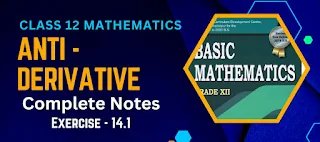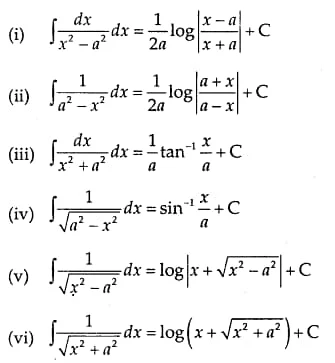# Antiderivative Class 12 Mathematics Solutions | Exercise - 14.1Antiderivative Class 12 Mathematics Solutions according to the updated syllabus of 2080. You'll get the complete solution of exercise class 12 antiderivatives chapter first exercise. You can also download the solutions PDF if you want to view them offline then you are offline.

## Chapter - 14  Antiderivative

In class 12 mathematics antiderivatives chapter, we will study about antiderivative of standard integrals, some integrals reducible to standard forms and integration of Rational fractions. We will discuss about them separately in different exercise.

Many people were demanding the handwritten notes of antiderivative chapter as we had provided the notes of other chapter  such as correlation and regression. So we are here with the complete solutions of antiderivative chapter.

### Antiderivative of standard integrals

The subject of integration can be treated in two different points of view. Actually it has developed in course of evaluation of the area under a plane curve and has been interpreted as a limit of a sum when the number of terms in the sum tends to infinity and each term tends to zero.

The other interpretation of integration as the inverse of differentiation came some time later. Both points of view are identical. The equivalence of these two view-points has been established in what is known as the Fundamental Theorem of Integral Calculus.

At the elementary stage, it is better to introduce the subject of integration as the inverse of differentiation, keeping in reserve the other one to use in some application. For a given function f(x) if there exists a function F(x) such that;

(dF(x))/(dx) = f(x)
then F(x) is said to be an integral of f(x) with respect to x. Symbolically, we write
∫f(x) dx = F (x)

The process of finding the integral of a function f(x) is called th function f(x) which has been integrated is called the integrar.

Exercise - 14.1

This PDF will provide you each and every question solutions from the 1st exercise of class 12 antiderivatives chapter. If you want the solutions of other exercises then you can click on the respective exercises from above button.

Note: Scroll the PDF to view all solutions

### Standard Integrals (I)

In this section, we discuss some integrals which are directly related to standard differentiation formulae and so may be considered as some of fundamental integrals.#### Some Integrals Reducible to Standard Forms

The integrals of the forms ∫1/(a * x² + bx + c) dx and (dx)/(sqrt(a * x² + bx + c)) can now be easily evaluated by converting them into above standard integrals as illustrated in the worked out examples below.

The other forms of integrals which can be reduced to the standard integrals are;

## Some Important Questions

In every maths exam, you'll be asked a defining type of question. It is not sure, from which chapter will the question come in your exam. So, here I'll provide you some of the possible questions that may be asked from antiderivatives chapter. Please note that all these questions are just predicted one and has no any guarantee to appear in examination.

### 1. What is antiderivative Class 12?

In calculus, the antiderivative of a function f(x) is a function F(x) whose derivative is equal to f(x). In other words, if F'(x) = f(x), then F(x) is an antiderivative of f(x).

Antiderivatives are also known as indefinite integrals. The process of finding an antiderivative is called integration. The symbol used to represent an antiderivative is the integral symbol ∫.

In Class 12, students will learn about integration techniques such as substitution, integration by parts, and partial fractions. They also learn about definite integrals, which involve finding the area under a curve between two limits.

### 2. What is antiderivative vs integral?

Antiderivative and integral are related concepts in calculus, but they are not the same thing.

An antiderivative of a function f(x) is a function F(x) whose derivative is equal to f(x). In other words, if F'(x) = f(x), then F(x) is an antiderivative of f(x). Antiderivatives are also known as indefinite integrals and are represented by the integral symbol ∫.

On the other hand, an integral is a mathematical tool used to find the area under a curve between two limits. The integral of a function f(x) over an interval `[a, b]` is denoted by ∫[a, b] f(x) dx. The result of this integration is a definite integral, which represents the area between the curve and the x-axis over the interval [a, b].

In other words, an antiderivative is a function whose derivative is equal to the original function, while an integral is a tool used to find the area under a curve. The two concepts are related in that the process of finding an antiderivative is called integration, and the integral of a function is related to its antiderivative through the fundamental theorem of calculus.

### 3. What is the antiderivative commonly called?

The antiderivative is commonly called the indefinite integral. This is because the antiderivative of a function f(x) is a family of functions that differ by a constant, and therefore cannot be expressed as a single definite value. The symbol used to represent an antiderivative is the integral symbol ∫, without any limits of integration. In contrast, the definite integral is used to find the area under a curve between two limits and is represented by the integral symbol ∫ with limits of integration.

No Comment